# Three-phase Transformer Connections (Wiring Diagrams Included)

Contents

## What is a Transformer Connection?

The primary and secondary winding of a three-phase transformer can be connected in different ways according to available terminals and the desired application.

A three-phase transformer is constructed by three single-phase transformers on separate cores, or on one combined core. But in most cases, the three-phase winding is placed on one core that provides an economic connection of copper windings and iron core.

In a power system network, power is generated, transmitted, and distributed in three-phase power. Therefore, to increase or decrease voltage levels in a three-phase system, we need three-phase transformers.

## Three-phase Connections

There are two methods to connect three-phase windings.

• Star connection
• Delta connection

### Star Connection

In star connection, one terminal of all three windings is connected and makes a star point or neutral point. Neutral terminal is taken from this point.

The second terminal of all windings is taken out, and supply is given to these terminals. Due to the shape of this configuration, it is known as star connection or Y-connection, or Wye-connection.

The connection diagram of the three-phase star connection is shown in the figure below.

### Delta Connection

In delta connection, all three windings are connected in series and make a triangular shape. The supply is given to three junction points.

The shape of this configuration looks like Delta (∆). Therefore, it is known as delta connection or ∆-connection. In some cases, this connection is also known as a mesh connection.

The connection diagram of the delta connection is as shown in the figure below.

## Factors Affecting Choice of Connection

The factors affecting the choice of connection are listed below.

• Availability of neutral connection for grounding and load connection.
• Availability of a path for zero-sequence current and third harmonic current.
• Voltage stress and insulation to the ground.
• Parallel operation with another transformer.
• Economic consideration.
• Operation of the transformer under fault condition.

## Three Phase Voltages and Currents in Star and Delta Connection

In the connection diagram, we have to consider line-to-line voltage-current and line-to-neutral voltage-current. Line-to-line voltage is known as line voltage, and line-to-neutral voltage is known as phase voltage.

In star connection, a line-to-line current is the same as a line-to-neutral current.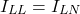To calculate voltage in star connection, we consider a three-phase four-wire configuration as shown in the above star connection figure. According to Kirchhoff’s law, we can find that the line-to-line voltage is √3 times line-to-neutral voltage.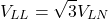In delta connection, line-to-line voltage is the same as line-to-neutral voltage.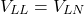## Three Phase Transformer Connections

A three-phase transformer consists of two three-phase windings; primary winding and secondary winding.

Primary winding and secondary winding can be connected as star or delta connections. According to the application of a transformer, the primary winding and secondary winding can be connected in four possible configurations.

• Delta-Delta (Δ-Δ)
• Star-Star (Y-Y)
• Delta-Star (Δ-Δ)
• Star-Delta (Y-Δ)

In the above configurations, the first configuration is for primary winding and the second configuration is for the secondary winding.

### Delta-Delta (Δ-Δ) Connection

In the delta-delta transformer connection, primary and secondary are connected in the Delta connection. The connection diagram of this configuration is shown in the figure below.

The primary winding is noted as A1A2, B1B2, and C1C2. The secondary winding is noted as a1a2, b1b2, and c1c2.

Terminals A1 and a1 have the same polarity. And primary winding A1A2 corresponds to secondary winding a1a2.

Phasor diagram of lagging power factor cos ф is shown in the figure below. In the phasor diagram, magnetizing current and voltage drops in impedance are neglected.

In delta-delta configuration, line voltage and phase voltage are same in magnitude on primary and secondary winding. The primary line-to-line voltage (VAB, VBC, VCA) are in phase with the secondary voltage (Vab, Vbc, Vca). And the voltage ratio is equal to the turns ratio of transformer.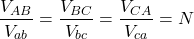The line current is √3 times phase current under balance condition. When magnetizing current is neglected, the current ratios is;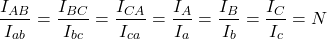In this type of connection, the primary and secondary winding voltage is in phase. Therefore, this connection is also known known as 0˚ connection.

If the connection of secondary winding is reversed, we obtain a 180˚ phase difference between primary and secondary winding. And this connection is known as a 180˚ connection.

The wiring diagram and phasor diagram of a 180˚ connection are as shown in the figure below.

In this connection, secondary terminal b1c2, c2a2, and a1b2 are connected. We can see in the phasor diagram that the secondary voltages are in the opposite phase to the primary voltage.

The advantages of delta-delta connection are;

• This connection can be used for balanced and unbalanced loading conditions.
• The third harmonic is present in this system, but it circulates in the close path and does not appear in the output voltage.
• The main advantage of this system is that if one phase fails to operate, the transformer will run on the other two phases. This system is known as open delta connection or V-V connection.

The disadvantage of the delta-delta connection is that the neutral point is not available in the system. Hence, a delta-delta connection is useful when primary or secondary winding does not require a neutral terminal.

### Star-Star (Y-Y) Connection

In a star-star connection, the primary winding and secondary winding are both connected in a star connection. The connection diagram of the star-star connection is shown in the figure below.

The phase current is equal to the line current in the star-star connection, and both currents are in phase. The line voltage is √3 times phase voltage. The phasor diagram of this connection is similar to the delta-delta connection.

For ideal transformer, the voltage ratio is;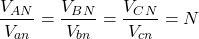And the current ratio is;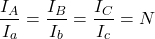When the load is unbalanced, the phase voltage become severely unbalanced. Because the neutral is not provided in star-star connection and this system is not useful with unbalanced load.

The main disadvantage of star-star connection is that it is highly affected by the magnetizing current.

In a transformer, the magnetizing current contains third harmonics, and it is non-sinusoidal in shape. To produce sinusoidal flux in a transformer, it is necessary to provide another path to the magnetizing current.

But in the case of star-star connection, magnetizing current of three-phase windings is equal in magnitude and in-phase with each other. Hence, it will be additive, and the sum at the star point is not zero.

In an ungrounded system, there is no path for the magnetizing current, and it will change the shape of the flux waveform. And this flux will produce voltage containing third harmonics on both the primary and secondary sides of a transformer.

This voltage will add to the fundamental voltage waveform, and it produces two times more peak voltage than the normal value.

The problem of unbalanced and third harmonics problem in star-star connection is solved by the below methods.

#### Solid Grounding of Neutral

If a solid grounding s provided between the star point of the primary transformer and the neutral point of an alternator, it will provide a path for the magnetizing current in the neutral wire. And it will not produce a large voltage in a transformer.

The neutral wire also provides a path for the unbalance current produced by the unbalanced loading condition.

In neutral wire, triple frequency current will pass. So, this wire may interfere with the communication circuit.

#### Provide Tertiary Winding

If it is necessary not to use neutral wire with a transformer, an extra winding is placed in the primary and secondary winding. This winding is known as tertiary winding.

It is connected in Delta connection. Therefore, the entire system will become star-delta-star (Y-∆-Y) system.

### Delta-Star (Δ-Y) Connection

In a Delta-star connection of a three-phase transformer, the primary winding is connected in Delta connection, and secondary winding is connected in star connection.

The connection diagram of a Delta-star configuration is as shown in the figure below.

The primary winding is connected in a Delta connection. Hence, in the primary winding, the line voltage is equal to phase voltage.

The secondary winding is connected in star connection. Hence, in the secondary winding, the line voltage is √3 times phase voltage.

Therefore, the voltage ratio of this configuration is;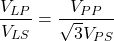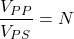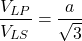The phasor diagram of delta-star connection for lagging power factor and balanced load condition is as shown in the figure below.

As shown in the phasor diagram, secondary phase voltage VaN leads to primary voltage VAN by 30˚. Similarly, secondary phase voltage VbN and VcN lead to primary voltage VBN and VCN, respectively. This connection is also known as the +30˚ connection.

If we reverse the connection on either side, we can get the secondary voltage lag primary voltage by 30˚. And this type of connection is known as -30˚ connection. The connection diagram is shown in the figure below.

### Star-Delta (Y-Δ) Connection

In a star-delta connection, the primary winding of a transformer is connected in a star configuration, and the secondary winding is connected in a delta configuration. The connection diagram of the star-delta configuration is shown in the figure below.

As the primary winding is connected in star, the primary line voltage equals √3 times phase voltage. And the secondary winding is connected in delta. So, in the secondary winding, the line voltage is equal to phase voltage.

The voltage ratio in this configuration is;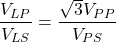The phasor diagram of this system can be drawn similar to the delta-star connection. In this system, the phase shift is 30˚ lead between line voltages. This connection is also known as the +30˚ connection.

If we reverse the connection, we can get a 30˚ lag phase shift. And this connection is known as -30˚ connection. The connection diagram of this system is shown in the figure below.

The star-delta simple obtain by interchanging the primary and secondary delta-star connection. Star-delta or delta-star connection can be used with unbalanced load and third harmonics.

The delta connection of Y- Δ and Δ-Y assures balanced voltage on the Y side and provides a path to flow the third harmonics and their multiples without using neutral wire.

## Open Delta Connection (V-V Connection)

In a Delta-delta system, one transformer is damaged or accidentally open, and the remaining two transformers will continue to supply a three-phase system with reduced load capacity. This connection is known as Open Delta connection or V-V connection.

Therefore, in an open delta connection, two out of three transformers operate a three-phase load.

The connection diagram of this system is shown in the figure below.

The applied voltage in primary is; VAB, VBC, and VCA. The voltage induced in secondary is; Vab and Vbc. There is no winding between point a and c. But a potential difference is created between points a and c.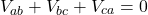(1)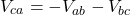Let say, line voltage in primary side is VP and secondary side VS.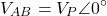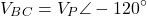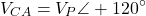To calculate the secondary side voltage, we assume zero leakage impedance in transformer.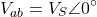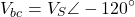Substitute these values in equation-1.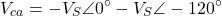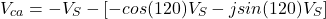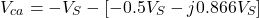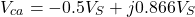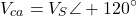Hence, it is proved that the voltage across points a and c is the same as secondary line voltage and 120˚ apart in time. Therefore, the applied voltage is a balanced three-phase voltage on the secondary side if we consider zero leakage impedance.

Now, in delta-delta connection, three transformers are connected, operating on 100% loading conditions. But in the case of an open delta connection, two transformers are connected.

It seems in open delta condition; we can connect 66.6% load. But this will not happen in this condition.

Suppose V2B and I2B are rated secondary phase voltage and phase current, respectively. And in delta connection, phase voltage and line voltage are the same and line current is √3 times phase current.

In close delta-delta condition,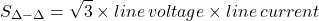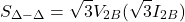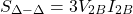Now, in open delta condition, one transformer is removed. Therefore, the line current I2B is equal to the rated secondary current of a transformer.

In open delta condition,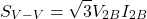Take a ratio of VA load for both conditions;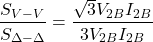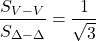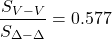So, we can see that, in open delta condition, we can connect 57.7% load of delta-delta condition.

Percentage increase in each transformer of V-V connection;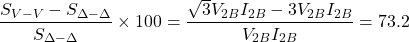Hence, each transformer in V-V connection is overloaded by 73.2%. Therefore, the load should be reduced in case of open delta condition. Otherwise, the transformer windings may overheat and in worst condition, it may breakdown.

## Scott Connection

Scott connection is used to connect two single-phase transformers to perform three-phase to two-phase conversion or vice versa.

In Scott connection, two transformers are connected electrically. But these transformers are not connected magnetically.

One transformer is the main transformer, and the second is known as an auxiliary transformer.

The connection diagram of Scott’s connection is as shown in the figure below.

Three-phase terminals are A, B, and C. The main transformer is a center-tapped transformer. And it is connected across B and C terminals. Tapping is taken from point D.

The primary winding is connected across B and C terminals, and the secondary winding is connected across a1a2.

The auxiliary transformer is connected between line terminal A and center tapped terminal D. The primary winding of an auxiliary transformer is connected between terminals A and C, and the secondary winding is connected between b1 and b2.

Three-phase line voltages (VAB, VBC, and VCA) are balanced. The phasor diagram of this system is shown in the figure below.

VBC is taken as a reference voltage.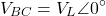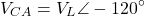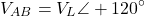D point divides primary winding BC into two equal parts. Hence, the number of turns in portion BD equals the number of turns in portion DC.

Let say the total number of turns in the primary winding of the main transformer is TP. Then, the number of turns in BD and DC is TP/2.

The voltage across BD and DC portion is same and it is half of voltage across BC.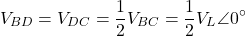The voltage between A and D is;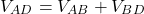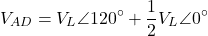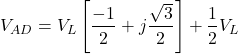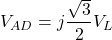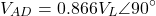Therefore, the voltage across a primary winding of an auxiliary transformer is 0.866 times of the main transformer, and the electrical angle between both voltages is 90˚.

The applied primary voltage of an auxiliary transformer is VAD, and the secondary voltage of an auxiliary transformer is V2a.

The secondary auxiliary voltage (V2a) will lead the secondary main voltage (V2m) by 90˚, as shown in the figure below.

The voltage per turn should be the same for the same flux in each transformer. Hence, to keep voltage per turn same in primary of main transformer and primary of an auxiliary transformer, we need to set 0.866TP turns in primary of an auxiliary transformer.

So, the secondary voltage of both transformers has equal voltage ratings. V2a and V2m are equal in magnitude and 90˚ apart. Therefore, it results in a balanced two-phase system.

Want To Learn Faster? 🎓
Get electrical articles delivered to your inbox every week.
No credit card required—it’s 100% free.